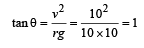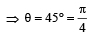Courses

# Test: Laws Of Motion 1 - From Past 28 Years Questions

## 14 Questions MCQ Test Physics 28 Years Past year papers for NEET/AIPMT Class 11 | Test: Laws Of Motion 1 - From Past 28 Years Questions

Description
This mock test of Test: Laws Of Motion 1 - From Past 28 Years Questions for NEET helps you for every NEET entrance exam. This contains 14 Multiple Choice Questions for NEET Test: Laws Of Motion 1 - From Past 28 Years Questions (mcq) to study with solutions a complete question bank. The solved questions answers in this Test: Laws Of Motion 1 - From Past 28 Years Questions quiz give you a good mix of easy questions and tough questions. NEET students definitely take this Test: Laws Of Motion 1 - From Past 28 Years Questions exercise for a better result in the exam. You can find other Test: Laws Of Motion 1 - From Past 28 Years Questions extra questions, long questions & short questions for NEET on EduRev as well by searching above.
QUESTION: 1

### A block of mass m is placed on a smooth wedge of inclination θθ. The whole system is accelerated horizontally so that the block does not slip on the wedge. The force exerted by the wedge on the block will be (g is acceleration due to gravity)

Solution: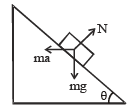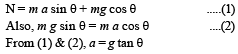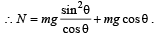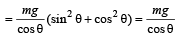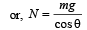QUESTION: 2

### 300 J of work is done in sliding a 2 kg block up an inclined plane of height 10 m. Taking g = 10 m/s2, work done against friction is 

Solution: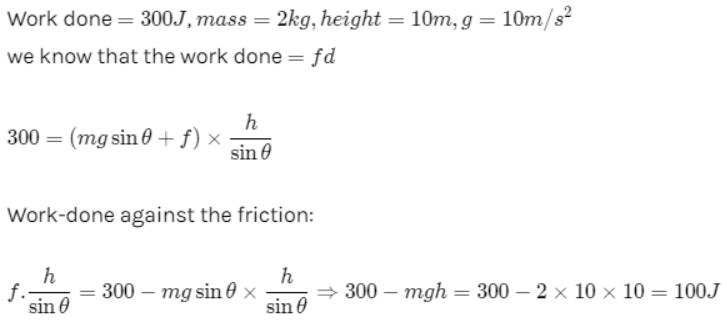QUESTION: 3

### A block  B is pushed momen tarily along  a horizontal surface  with an initial velocity V. If μ is the coefficient of sliding friction  between B and the surface, block B will come to rest after a time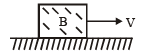Solution:

Friction is the retarding force for the block F = ma = μR = μmg
Therefore, from the first equation of motion v = u – at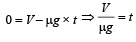QUESTION: 4

Sand is being dropped on a conveyor belt at the rate of M kg/s. The force necessary to keep the belt moving with a constant velocity of v m/s will be

Solution:

Taking derivative of mass. We cant take derivative of velocity hence it is constant as it is mentioned in the question.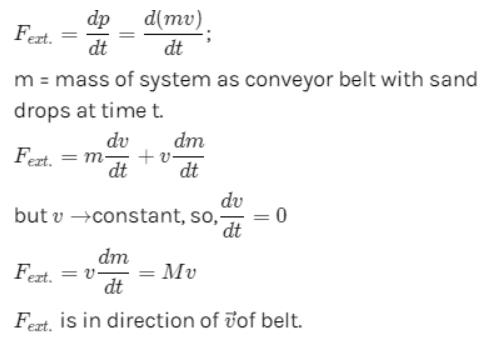QUESTION: 5

A body under the action of a force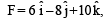acquires an acceleration of 1 m/s2. The mass of this body must be

Solution: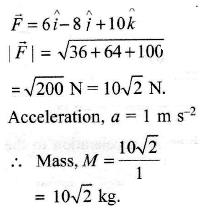QUESTION: 6

The mass of a lift is 2000 kg. When the tension in the supporting cable is 28000 N, then its acceleration is: 

Solution:

Net force, F = T – mg
ma = T – mg
2000 a = 28000 – 20000 = 8000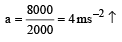QUESTION: 7

A person of mass 60 kg is inside a lift of mass 940 kg and presses the button on control panel.The lift starts moving upwards with an acceleration  1.0 m/s2. If g = 10 ms–2, the tension in the supporting cable is 

Solution: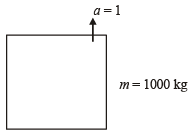Total mass = (60 + 940) kg = 1000 kg
Let T be the tension in the supporting cable, then T – 1000g = 1000 × 1
⇒ T = 1000 × 11 = 11000 N

QUESTION: 8

A body of mass M hits normally a rigid wall with velocity V and bounces back with the same velocity. The impulse experienced by the body is

Solution:

Impulse experienced by the body = change in momentum = MV – (–MV)
= 2MV.

QUESTION: 9

A conveyor belt is moving at a constant speed of 2m/s. A box is gently dropped on it. The coefficient of friction between them is µ = 0.5. The distance that the box will move relative to belt before coming to rest on it taking g = 10 ms–2, is [2011M]

Solution:

Frictional force on the box f = μmg
∴ Acceleration in the box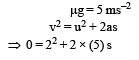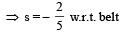⇒ distance = 0.4 m

QUESTION: 10

A car of mass 1000 kg negotiates a banked curve of radius 90 m on a frictionless road. If the banking angle is 45°, the speed of the car is : 

Solution:

For banking tan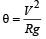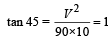V = 30 m/s

QUESTION: 11

A stone is dropped from a height h. It hits the ground with a certain momentum P. If the same stone is dropped from a height 100% more than the previous height, the momentum when it hits the ground will change by : [2012M]

Solution:

Momentum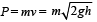(v2 = u2 + 2gh; Here u = 0)

When stone hits the ground momentum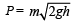when same stone dropped from 2h (100% of initial) then momentum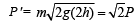Which is changed by 41% of initial.

QUESTION: 12

A car of mass m is moving on a level circular track of radius R. If μs represents the static friction between the road and tyres of the car, the maximum speed of the car in circular motion is given by :

Solution:

For smooth driving maximum speed of car v then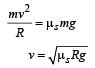QUESTION: 13

Three blocks with masses m, 2 m and 3 m are connected by strings as shown in the figure.After an upward force F is applied on block m, the masses move upward at constant speed v.What is the net force on the block of mass 2m? (g is the acceleration due to gravity) [NEET 2013]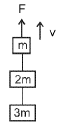Solution: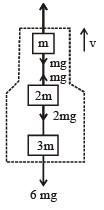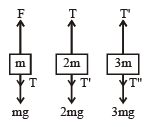From figure F = 6 mg, As speed is constant, acceleration a = 0
∴ 6 mg = 6ma = 0, F = 6 mg
∴ T = 5 mg , T' = 3 mg T" = 0
Fnet on block of mass 2 m = T – T' – 2 mg = 0
ALTERNATE :
v  = constant  so, a = 0, Hence, Fnet = ma = 0

QUESTION: 14

A car is moving in a circular horizontal track of radius 10 m with a constant speed of 10 m/s. A bob is suspended from the roof of the car by a light wire of length 1.0 m. The angle made by the wire with the vertical is [NEET Kar. 2013]

Solution:

Given; speed = 10 m/s; radius r = 10 m

Angle made by the wire with the vertical